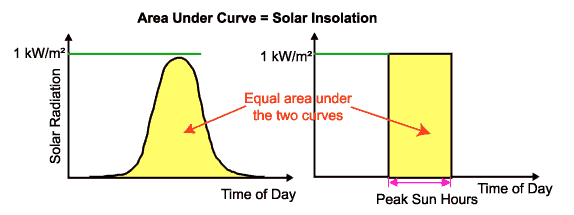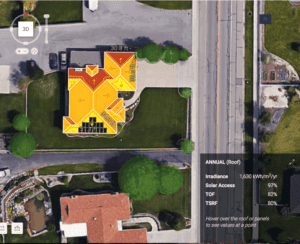# How to Size a PV System from an Electricity Bill

## Sizing a PV System from an Electricity Bill

An electricity bill typically reveals information about a residential or commercial customer’s total monthly energy consumption (as we discussed in the previous article in this seriesReading Your Electricity Bill: A Beginner’s Guide). From this value alone, it is possible to approximate the required size of a PV system that offsets monthly energy usage.

Take a hypothetical monthly energy consumption of 500 kilowatt-hours, which is on the lower end for a household in California. Assuming there are 30 days in a month, an average daily energy use value can be reached by dividing the monthly use by 30.

Daily Energy Use=Monthly Energy Use/Days in Month
=500kWh/mo / 30days/mo=16.7kWh/day

Next, insolation values are needed. As mentioned in The Beginner’s Guide to Solar Energy, insolation values are reported in kWh/m2-day. Since a “full-sun’s” worth of incoming solar energy is approximated as 1 kW/m2, insolation values reported in kWh/m2-day approximate the hours of full-sun equivalent that a location receives over the course of a day.Figure1. Visualization of how total solar insolation received over the course of a day (left) can be represented by number of full-sun hours (right). Source: pveducation.org

For a Palo Alto home, the average daily irradiance value is 5.2 kWh/m2-day. By dividing the daily energy usage by hours a day of full sun, the power output required by the PV system is calculated.

Power Output=Daily Energy Use * Daily hours of full sun
=16.7kWh/day * 5.2hours/day=3.21kWFigure 2. The Palo Alto home used for this PV system sizing exercise. Source: Aurora Solar

This would be the size of the PV system required, if our system was 100% efficient. However, that is not the case because all PV systems have a corresponding derating factor that takes into account the inefficiencies of the overall system, such as soiling of the panels and imperfect electrical connections.

According to the National Renewable Energy Laboratory’s PVWatts calculator, a typical derate factor is 0.84. For the sake of this calculation, we assume the derate factor be 80%, or 0.8. In order to determine the size of the PV system, divide the required power output by the derate factor.

PV System Size=Power Output / Derate Factor
=3.21kW / 0.8=4.01k

From this analysis, the approximate size of a PV system required to completely offset the average monthly energy usage of a 500 kWh/month home in Palo Alto would be about 4 kW.## Comparing the PV Size Estimation to a Simulated Result

Since this is a rough estimate, how does it compare against an actual, comprehensive design for a home with the same characteristics?

Using the same conditions as above, a PV system design software found that the required system size to be 4 kW, which is almost identical to the answer from the estimation conducted above.

Although the answers are very close, it’s important to note that this may not always be the case. For instance, when there is shading on the panels, a significant reduction in power output can occur. Although a shading term is included when calculating a derate factor, it can fail to accurately capture the effect that shading has on a PV system’s power output. Therefore, expect the results to be less close when modeling a location with shading.

### About Solar PV Education 101

How to Size a PV System from an Electricity Bill is part of Solar PV Education 101, a six-article series that serves as an introductory primer on the fundamentals of solar PV for beginners.